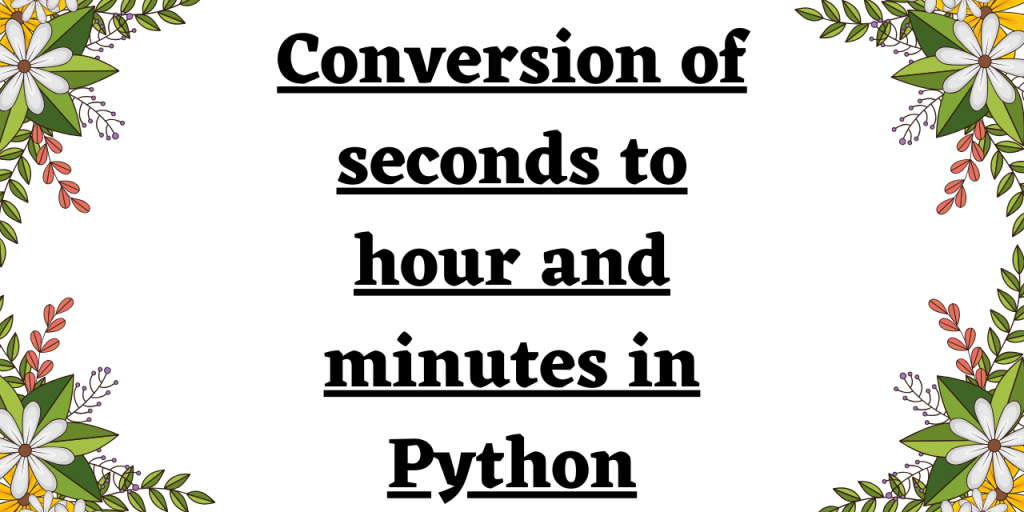# Python Program to Convert Seconds to Hours and MinutesSometimes we have to convert seconds to hours and minutes in Python. It’s mostly required when we are storing data in the form of a timestamp and we have to display it properly in minutes and seconds format. In this article, we will look at the Python program to convert seconds to hours and minutes.

## Conversion Rules for Different Time Units

Every day consists of 24 hours. Every hour has 60 minutes and every minute has 60 seconds. So, an hour has 3,600 seconds and a day consists of 86,400 seconds.

There are different ways to convert seconds to minutes and minutes to hours in Python.

## Method 1: Defining a Python function to convert seconds to hours and minutes

We can write a custom Python function to convert seconds value into hours and minutes.

Initially, we convert the input seconds value according to the 24-hour format.

seconds = seconds % (24*3600)

Since 1 hour is equivalent to 3600 seconds and 1 minute is equivalent to 60 seconds, we follow the below logic to convert seconds to hours and minutes.

hour = seconds//3600

min = seconds // 60

Example:

```def time_conversion(sec):
sec_value = sec % (24 * 3600)
hour_value = sec_value // 3600
sec_value %= 3600
min = sec_value // 60
sec_value %= 60
print("Converted sec value in hour:",hour_value)
print("Converted sec value in minutes:",min)

sec = 50000
time_conversion(sec)
```

Output:

```Converted sec value in hour: 13
Converted sec value in minutes: 53
```

## Method 2: Python time module to convert seconds to minutes and hours

Python time module contains time.strftime() function to display the timestamp as a string in a specified format by passing the format code as an argument.

The time.gmtime() function is used to convert the value passed to the function into seconds. Further, `time.strftime() function` displays the value passed from `time.gmtime() function` to hours and minutes using the specified format codes.

Example:

```import time
sec = 123455
ty_res = time.gmtime(sec)
res = time.strftime("%H:%M:%S",ty_res)
print(res)
```

Output:

```10:17:35
```

## Method 3: The Naive method

Example:

```sec = 50000
sec_value = sec % (24 * 3600)
hour_value = sec_value // 3600
sec_value %= 3600
min_value = sec_value // 60
sec_value %= 60
print("Converted sec value in hour:",hour_value)
print("Converted sec value in minutes:",min_value)

```

Output:

```Converted sec value in hour: 13
Converted sec value in minutes: 53
```

## Method 4: Python datetime module

Python datetime module has various in-built functions to manipulate date and time. The `datetime.timedelta() function` manipulates and represents the data in a proper time format.

Example:

```import datetime
sec = 123455
res = datetime.timedelta(seconds =sec)
print(res)
```

Output:

```1 day, 10:17:35
```

## Summary

Python provides many modules to convert seconds to minutes and hours. We can create our own function or use the time and datetime modules.